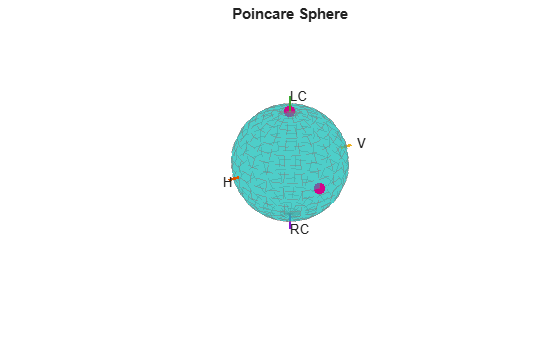Documentation

stokes

Stokes parameters of polarized field

Description

example

G = stokes(fv) returns the four Stokes parameters G of a polarized field or set of fields specified in fv. The field should be expressed in terms of linear polarization components. The expression of a field in terms of a two-row vector of linear polarization components is called the Jones vector formalism.

example

stokes(fv) displays the Stokes parameters corresponding to fv as points on the Poincare sphere.

Examples

collapse all

Create a left circularly-polarized field. Convert it to a linear representation and compute the Stokes vector.

cfv = [2;0];
fv = circpol2pol(cfv);
G = stokes(fv)
G = 4×1

4.0000
0
0
4.0000

Display points on the Poincare sphere for a left circularly-polarized field and a 45° linear polarized field.

fv = [sqrt(2)/2, 1; sqrt(2)/2*1i, 1];
G = stokes(fv)
G = 4×2

1.0000    2.0000
0         0
0    2.0000
1.0000         0

stokes(fv);The point at the north pole represents the left circularly-polarized field. The point on the equator represents the 45° linear polarized field.

Input Arguments

collapse all

Field vector in its linear polarization representation specified as a 2-by-N complex-valued matrix or in its linear polarization ratio representation specified as a 1-by-N complex-valued row vector. If fv is a matrix, each column of fv represents a field in the form [Eh;Ev], where Eh and Ev are its horizontal and vertical linear polarization components. The expression of a field in terms of a two-row vector of linear polarization components is called the Jones vector formalism. If fv is a vector, each entry in fv is contains the polarization ratio, Ev/Eh.

Example: [sqrt(2)/2*1i; 1]

Data Types: double
Complex Number Support: Yes

Output Arguments

collapse all

G contains the four Stokes parameters for each polarized field specified in fv. The Stokes parameters are computed from combinations of intensities of the field:

• G0 describes the total intensity of the field.

• G1 describes the preponderance of horizontal linear polarization intensity over vertical linear polarization intensity.

• G2 describes the preponderance of +45° linear polarization intensity over -45° linear polarization intensity.

• G3 describes the preponderance of right circular polarization intensity over left circular polarization intensity.

 Mott, H., Antennas for Radar and Communications, John Wiley & Sons, 1992.

 Jackson, J.D. , Classical Electrodynamics, 3rd Edition, John Wiley & Sons, 1998, pp. 299–302.

 Born, M. and E. Wolf, Principles of Optics, 7th Edition, Cambridge: Cambridge University Press, 1999, pp 25–32.